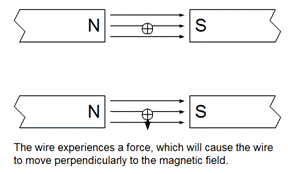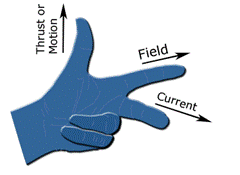Experiments with magnets and our surroundings

Twelve Fundamentals of Magnetism

12.   Motor Action

http://en.wikipedia.org/wiki/Lorentz_force
b. What does this mean?

When a current carrying wire is within a magnetic field, a force that is perpendicular to both the magnetic field and the current is impressed upon the wire.This force is called the Lorenz force after Hendrik Antoon Lorentz who first formulated it. It was discovered either in 1865 (by James Clerk Maxwell) or in 1889 (by Oliver Heavyside).  It was later verified by JJ Thompson in 1897.

d. How much force is created?

The magnitude of the force, F = B*L*i

i. F is the Lorenz force, Newtons

ii. B is the magnetic flux density, T, within which the wire is immersed

iii. L is the length of the wire in meters which is immersed within the magnetic flux density

iv. i is the current, Amperes, flowing within the wire

Another very simple equation!  The force on the wire is only affected by the strength of the magnetic field, the amount of current flowing, and the length of wire within the magnetic field.

e. What if the wire is constrained to move about an axis within the magnetic field?

If the wire is forced to move in a circle around an axis, a torque is produced where T = F*r

Where:

i. T is the torque in Newton-meters

ii. F is the force on the wire, Newtons

iii. r is the radius of the circle, meters

f. What direction is the force on the wire?

To determine the direction of the force on the wire, the Left Hand Rule is used (LHR).

i. The thumb of the left hand points in the direction of motion of the wire with respect the magnetic flux due to the force exerted upon it

ii. The first (index) finger of the left hand points in the direction of the magnetic flux (or think of this as the first finger pointing in the direction of the field)

iii. The second finger of the left hand points in the direction of the current that is flowing within the wire

iv. This motor action is the only time we use the Left-Hand-Rule.